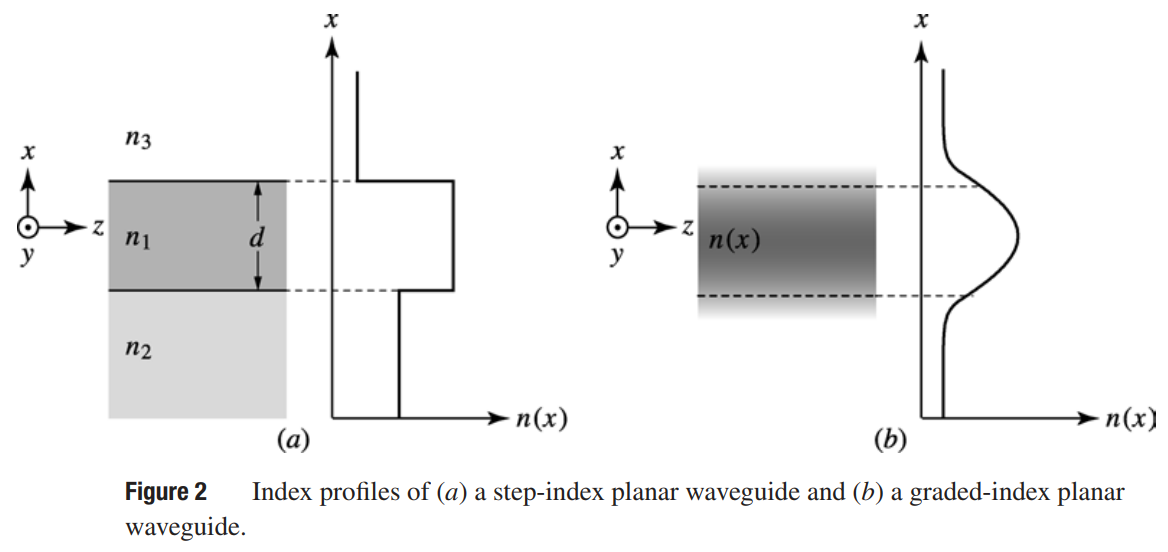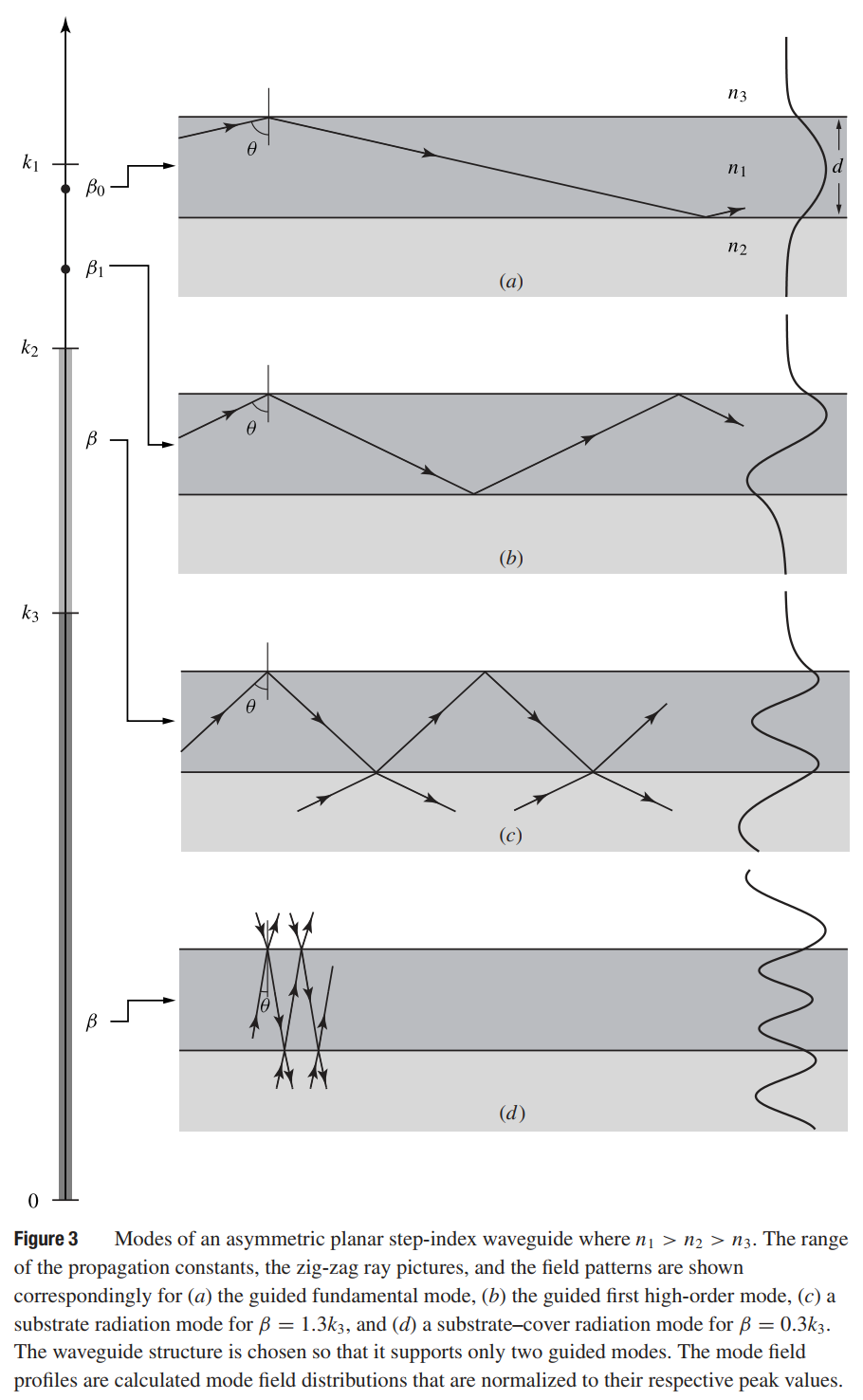# Waveguide Modes

This is a continuation from the previous tutorial - Material Dispersion.

Optical waveguides are the basic elements for confinement and transmission of light over various distances, ranging from tens or hundreds of micrometers in integrated photonics to hundreds or thousands of kilometers in long-distance fiber-optic transmission. They are used to connect various photonic devices. In many devices, they form important parts or key structures, such as the waveguides providing optical confinement in semiconductor lasers. Furthermore, they form important active or passive photonic devices by themselves, such as waveguide couplers and modulators. In this part, we consider the basic characteristics of linear, lossless dielectric waveguides.

The basic structure of a dielectric optical waveguide consists of a longitudinally extended high-index optical medium, called the core, which is transversely surrounded by low-index media, called the cladding. A guided optical wave propagates in the waveguide along its longitudinal direction. We consider a straight waveguide whose longitudinal direction is taken to be the z direction, as shown in figure 1(a). The characteristics of a waveguide are determined by the transverse profile of its dielectric constant ε(x, y)/ε0, which is independent of the z coordinate. For a waveguide made of optically isotropic media, we can simply characterize the waveguide with a single spatially dependent transverse profile of the index of refraction, n(x, y).

In a nonplanar waveguide of two-dimensional transverse optical confinement, the core is surrounded by cladding in all transverse directions, and n(xy) is a function of both x and y coordinates. The channel waveguides and circular optical fibers are such waveguides. In a planar waveguide that has optical confinement in only one transverse direction, the core is sandwiched between cladding layers in only one direction, say the x direction, with an index profile n(x), as shown in figure 1(b). The core of a planar waveguide is also called the film, while the upper and lower cladding layers are called the cover and the substrate, respectively. Optical confinement is provided only in the x direction by the planar waveguide shown in figure 1(b).A waveguide in which the index profile has abrupt changes between the core and the cladding is called a step-index waveguide, while one in which the index profile varies gradually is called a graded-index waveguide. Figure 2 shows examples of step-index and graded-index planar waveguides.Waveguide modes exist that are characteristic of a particular waveguide structure. A waveguide mode is a transverse field pattern whose amplitude and polarization profiles remain constant along the longitudinal z coordinate. Therefore, the electric and magnetic fields of a mode can be written in the following form:

$\tag{1}\mathbf{E}_\nu(\mathbf{r},t)=\pmb{\mathcal{E}}_\nu(x,y)\exp(\text{i}\beta_\nu{z}-\text{i}\omega{t})$

$\tag{2}\mathbf{H}_\nu(\mathbf{r},t)=\pmb{\mathcal{H}}_\nu(x,y)\exp(\text{i}\beta_\nu{z}-\text{i}\omega{t})$

where ν is the mode index, $$\pmb{\mathcal{E}}_\nu(x,y)$$ and $$\pmb{\mathcal{H}}_\nu(x,y)$$ are the mode field profiles, and βν is the propagation constant of the mode. For a waveguide of two-dimensional transverse optical confinement, there are two degrees of freedom in the transverse xy plane, and the mode index ν consists of two parameters for characterizing the variations of the mode fields in these two transverse dimensions. For example, ν represents two mode numbers, ν = mn with integral m and n, for discrete guided modes. For the planar waveguide shown in figure 1(b), the mode fields do not depend on the y coordinate. Thus, (1) and (2) are reduced to

$\tag{3}\mathbf{E}_\nu(\mathbf{r},t)=\pmb{\mathcal{E}}_\nu(x)\exp(\text{i}\beta_\nu{z}-\text{i}\omega{t})$

$\tag{4}\mathbf{H}_\nu(\mathbf{r},t)=\pmb{\mathcal{H}}_\nu(x)\exp(\text{i}\beta_\nu{z}-\text{i}\omega{t})$

respectively. In this case, ν consists of only one parameter characterizing the field variation in the x dimension.

To get a general idea of the modes of a dielectric waveguide, it is instructive to consider the qualitative behavior of an optical wave in the asymmetric planar step-index waveguide shown in figure 2(a), where n1 > n2 > n3. For an optical wave of angular frequency ω and free-space wavelength λ, the media in the three different regions of the waveguide define the following propagation constants:

$\tag{5}k_1=n_1\frac{\omega}{c},\;k_2=n_2\frac{\omega}{c},\;\text{and}\;\,k_3=n_3\frac{\omega}{c},$

where k1 > k2 > k3.

An intuitive picture can be obtained from studying ray optics by considering the path of an optical ray, or a plane optical wave, in the waveguide, as shown in the central column of figure 3 below. There are two critical angles associated with the internal reflections at the lower and upper interfaces:

$\tag{6}\theta_{c2}=\sin^{-1}\frac{n_2}{n_1}\qquad\text{and}\qquad\theta_{c3}=\sin^{-1}\frac{n_3}{n_1}$

respectively. We see that θc2 > θc3 because n2 > n3.The characteristics of the reflection and refraction of the ray at the interfaces depend on the angle of incidence θ and the polarization of the wave.

1. Guided modes. If θ > θc2 > θc3, the wave inside the core is totally reflected at both interfaces and is trapped by the core, resulting in guided modes. As the wave is reflected back and forth between the two interfaces, it interferes with itself. A guided mode can exist only when a transverse resonance condition is satisfied so that the repeatedly reflected wave has constructive interference with itself.

In the core region, the x component of the wavevector is k1cosθ for a ray with an angle of incidence θ, while the z component is βk1sinθ. The phase shift in the optical field caused by a round-trip transverse passage in the core of thickness d is 2k1dcosθ.

In addition, there are phase shifts φ2 and φ3 associated with the internal reflections at the lower and upper interfaces, respectively. These phase shifts can be obtained from the phase angle of rs in (147) for a TE wave and that of rp in (151) for a TM wave for a given θi = θ > θc2, θc3. [refer to the reflection and refraction tutorial].

Because φ2 and φ3 are functions of θ, the transverse resonance condition for constructive interference in a round-trip transverse passage is

$\tag{7}2k_1{d}\cos{\theta}+φ_2(\theta)+φ_3(\theta)=2m\pi$

where m is an integer. Because m can assume only integral values, only certain discrete values of θ can satisfy (7). This results in discrete values of the propagation constant βm for guided modes identified by the mode number m.

The guided mode where m = 0 is called the fundamental mode and those with m ≠ 0 are high-order modes.

Although the critical angles,  θc2 and θc3, do not depend on the polarization of the wave, the phase shifts, φ2 and φ3, caused by internal reflection at a given angle θ depend on the polarization. Therefore, TE and TM waves have different solutions for (7), resulting in different βm and different mode characteristics for a given mode number m.

For a given polarization, solution of (7) yields a smaller value of θ and a correspondingly smaller value of β for a larger value of m. Therefore, β0 for the fundamental mode has the largest value among the allowed values for β, and β0 > β1 > ..., as shown in figure 3(a) and 3(b).

2. Substrate radiation modes. When θc2 > θ > θc3, total reflection occurs only at the upper interface but not at the lower interface. As a result, an optical wave incident from either the core or the substrate can be refracted at the lower interface. This wave is not confined to the core, but is transversely extended to infinity in the substrate. It is called a substrate radiation mode. In this case, the angle θ is not dictated by a resonance condition like (7) but can assume any value in the range of θc2 > θ > θc3. As a result, the allowed values of β form a continuum between k2 and k3, and the modes are not discrete. These characteristics of a substrate radiation mode are illustrated in figure 3(c).

3. Substrate-cover radiation modes. When θc2 > θc3 > θ, there is no total reflection at either interface. In this case, an optical wave incident from either side is refracted at both interfaces, and it can transversely extend to infinity on both sides of the waveguide, resulting in substrate-cover radiation modes. These modes are not discrete, and the allowed values of β for them form a continuum between k3 and 0. These characteristics of a substrate-cover radiation mode are illustrated in figure 3(d).

In addition to the three types of modes discussed above, there are also evanescent radiation modes, which have purely imaginary values of β that are not discrete. Their fields decay exponentially along the z direction. Because the waveguide is lossless and does not absorb energy, the energy of an evanescent mode radiates away from the waveguide transversely. A lossless waveguide cannot generate energy, either. Therefore, the evanescent modes do not exist in perfect, longitudinally infinite waveguides. They exist at the longitudinal junctions or imperfections of a waveguide, as well as at the terminations of a realistic waveguide of finite length. In comparison, a substrate radiation mode or a substrate-cover radiation mode has a real β; hence, its energy does not decay as it propagates. In such a radiation mode, the power flowing away from the center of the waveguide in the transverse direction is equal to that flowing toward the center.

The approach of ray optics gives a very intuitive picture of the waveguide modes and their key characteristics. Nevertheless, this approach has many limitations. In more complicated waveguide geometries such as that of a circular fiber, the idea of using the resonance condition based on the total internal reflection to find the allowed values of β for the guided modes does not necessarily yield correct results. [For an excellent detailed discussion on this point, see Marcuse, D., Theory of Dielectric Optical Waveguides. Page 89.]

For a complete description of the waveguide fields, rigorous analyses using electromagnetic wave equations are required.

The next part continues with the Optical Waveguide Field Equations tutorial.